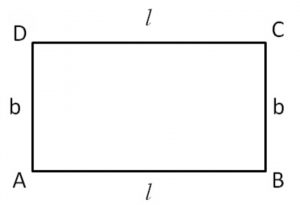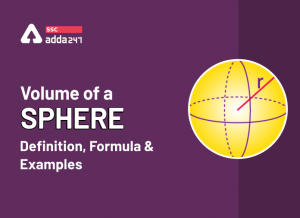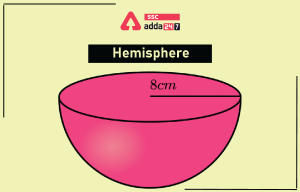Latest SSC jobs   »   Rectangles   »   Rectangles

# What is Rectangle? Definition, Area, Formula, Properties and Examples

## What is Rectangle?

A rectangle is a two-dimensional plane figure having four sides and four internal angles. The opposite sides and angles have an equal measure. It is defined as a type of quadrilateral having four sides and opposite sides and angles are equal and parallel to each other. The rectangular object is specified based on two parameters i.e. length and width. The longest side of the rectangle is considered as a length while the shortest side is taken as width.

In our daily life, we observe and use various rectangular-shaped objects such as Tables, books, boxes, Mobile phones, walls, Cricket pitches, TV or Computer Screens, furniture, beds, Almirah, Doors, etc. In this article, we will discuss the rectangle, its properties, the area of a rectangle, formulas, the perimeter of a rectangle, and some solved examples based on it to make a clear understanding of the concepts of the rectangle.

## Rectangle Definition

A rectangle is defined as any two-dimensional closed figure having four sides and the opposite sides and angles are equal. The opposite sides see parallel that they never meet with each other. In a rectangle, all the four internal angles are 90°. You can observe the rectangle its sides and angles in the figure shown below.In the above figure, a rectangle ABCD is shown having sides in which the length of sides AB = CD = l, AD = BC = b, and AD || BC, AB || CD. The values of internal angles are 90°.

## Area of Rectangle

The area of a rectangle is the amount of space occupied inside the four sides. Mathematically, the number of unit squares placed inside the rectangle gives the area of a rectangle. In our daily life, we observe many rectangular objects, and the need of finding their areas is also required. We can take the example of many rectangular objects like books, tables, laptop screens, CPUs, etc. The area of a rectangle depends on only two parameters like length and breadth. The area of the rectangle can be found using the formula shine below. The area of a rectangle is represented in terms of square units sq. m, sq. cm, or sq. mm.

Area of rectangle = length × breadth

A = l × B Square units

## Rectangle Shape

As we discussed already, the rectangle is a two-dimensional closed figure having four sides. The opposite sides of the rectangle are equal and parallel to each other and it has four internal angles which are equal to 90°. We observe many rectangular shapes such as walls, boxes, tables, beds, computer/laptop screens, etc.

## Rectangle Area Formula

The area of the rectangle is the space occupied inside the outer boundary of the rectangle. The rectangle area can be calculated by the product of length and breadth. Consider the length of a rectangle is l, the breadth of a rectangle is b, the d area of a rectangle is represented by A then the formula to calculate the area of a rectangle is the product of length and breadth. The area of a rectangle is represented in square units.

Area of rectangle = length × breadth

A = l × b square units

## Rectangle Area Using diagonals

The area of a rectangle can also be determined through diagonals as discussed below

• (Diagonal)2 = (Length)2 + (Width)2
• (Length)2 = (Diagonal)2 – (Width)2
• Length = √ (Diagonal)^2 – (Width)^2
• (Width)2 = (Diagonal)2 – (Length)2
• Width = √(Diagonal)^2 – (length)^2
• We know that the area of a rectangle is the product of length and breadth
• Area of rectangle = length × breadth
• Area of rectangle = length × √(Diagonal)^2 – (Length)^2
• or Area of rectangle = √ (Diagonal)^2 – (Width)^2 × width

## Rectangle Formula

The formula to calculate the area of a rectangle is discussed above in detail. The formula of the area of a rectangle is the multiplication of the magnitude of length and breadth of the rectangle. Let the length of the rectangle be L, breadth be W and the area of a rectangle is A. Then the formula of rectangle area can be represented as:

A = L × W square units

## Rectangle Perimeter Formula

The perimeter of a rectangle is the total distance of its outer boundaries. It is twice the sum of the length and breadth of the rectangle. The perimeter of a rectangle is a linear measurement of its outer sides and then it is added to get the value of a parameter of the rectangle. It is expressed in terms of units of length like m, cm, or mm.Consider a rectangle ABCD as shown above whose length is l and width is b then the perimeter of the rectangle ABCD is represented by

The perimeter of the rectangle = 2 (+ b)

## Rectangle Properties

A rectangle has many properties used in geometry and other applications through which it is specified. The important properties of the rectangle are listed below.

• A rectangle is a type of quadrilateral.
• Opposite sides of a rectangle are equal and parallel to each other
• The value of the interior angle of a rectangle at each vertex is 90°.
• The sum of all interior angles of rectangles is 360° (90°+90°+90°+90°).
• The diagonals of a rectangle bisect each other.
• The length of both diagonals of a rectangle is equal.
• The length of the diagonals can be found using the Pythagoras theorem. The length of the diagonal with sides a and b is, then diagonal = √( a2 + b2).
• Since the sides of a rectangle are parallel, it is also known as a parallelogram.
• All rectangles are parallelogram definitely but all parallelograms cannot be rectangles.

## Rectangle: Solved Examples

1. If the length of a rectangle is 8cm and the breadth measures 5cm. Find the area of a rectangle.

Solution: Given l = 8cm and b = 5cm

then the area of rectangle A = l × b

A = 8 × 5

A = 40 sq. cm

2. The breadth of the rectangle measures 20mm and the area of a rectangle is 220mm. Calculate the length of the rectangle.

Solution: Given b = 20mm, A = 220mm a, and l =?

We know that, the area of rectangle = length × breadth

A = l × b

220 = 20l

l = 220/20 = 11mm

3. Shyam has a rectangular photo frame that is 9 inches long and 5 inches wide. Help Shyam in finding the area of the photo frame?

Solution: We know the formula to calculate the area of a rectangle

Area of a Rectangle = (Length × Width).

Thus, the area of the rectangular frame = 9 × 5 = 45 square inches

Therefore, the area of the photo frame = 45 square inches

4. Find the perimeter and area of the rectangle o with a length of 17 cm and a breadth of 13 cm.

Solution:  Given length = 17 cm, breadth = 13 cm

The perimeter of the rectangle = 2 (length + breadth)

= 2 (17 + 13) cm

= 2 × 30 cm

= 60 cm

We know that the area of rectangle = length × breadth

= (17 × 13) cm2

= 221 cm2

5. Find the breadth of the rectangular plot of land whose area is 660 m2 and whose length is 33 m. Find its perimeter.
Solution: Given that A = 660m^2, and l = 33m

A = l × b

660 = 33 × b                                                                      b  = 20 m

Therefore, the perimeter of the rectangular plot = 2 (length +breadth)  = 2(33 + 20) m

= 2×53                                                                = 106m

6. Find the area of the rectangle if its perimeter is 48 cm and its breadth is 6 cm.

Solution: P = 2 (l + b)

Here, P = 48 cm; b = 6 cm

Therefore, 48 = 2 (l + 6)

⇒ 48/2 = l + 6

⇒ 24 = l + 6

⇒ 24 – 6 = l

⇒ 18 = l

Therefore, length = 18 cm

Now, the area of the rectangle = l × b = 18 × 6 cm2 = 108 cm2

7. Find the breadth and perimeter of the rectangle if its area is 96 cm22
and the length is 12 cm.
Solution: Given, that A = 96 cm2 and l = 12 cm

A = l × b

Therefore, 96 = 12 × b

96/12 = b

⇒ b = 8 cm

Now, P = 2 (l + b)

= 2 (12 + 8)

= 2 × 20

= 40 cm

8. The length and breadth of a rectangular courtyard are 75 m and 32 m. Find the cost of leveling it at the rate of $3 per m2. Also, find the distance covered by a boy to take 4 rounds of the courtyard. Solution: Length of the courtyard = 75 m The breadth of the courtyard = is 32 m The perimeter of the courtyard = 2 (75 + 32) m = 2 × 107 m = 214 m Distance covered by the boy in taking 4 rounds = perimeters of the courtyard = 4 × 214 = 856 m We know that area of the courtyard = length × breadth = 75 × 32 m22 = 2400 m22 For 1 m22, the cost of leveling =$3

For 2400 m22, the cost of leveling = $3 × 2400 =$7200

9. How many envelopes can be made out of a sheet of paper 100 cm by 75 cm, supposing 1 envelope requires a 20 cm by 5 cm piece of paper?

Solution: Area of the sheet = 100 × 75 cm2  = 7500 cm2

Area of envelope = 20 × 5 cm = 100 cm2

Number of envelopes that can be made = Area of sheet /Area of the envelope

= 7500/100 = 75 envelopes

10. A wire in the shape of a rectangle of length 35 cm and breadth of 18 cm is rebent to form a square. What will be the measure of each side?

Solution: The perimeter of the rectangle = 2 (35 + 18) cm

= 2 × 53

= 106 cm

The Perimeter of the square of side x cm = the, Therefore, perimeter of the  rectangle = Perimeter of Square

106 cm = 4x

⇒ x = 26.5

Therefore, each side of the square = 26.5 cm

### Rectangle: FAQs

Que.1 What is a rectangle?

Ans – A rectangle is a closed two-dimensional figure having four sides and four angles. The opposite sides are parallel and equal.

Que.2 Define the area of the rectangle?

Ans – The area of a rectangle is the region occupied under the boundary of the rectangle. It is a product of length and breadth.

Que.3 What is the perimeter of the rectangle?

Ans – The perimeter of a rectangle is the total length of sides of a rectangle. It is calculated by the formula 2(l+b).

Que.4 What are the diagonals of a rectangle?

Ans – The diagonals are the line joining the opposite vertex of the rectangle. It is equal in length.

#### Congratulations!General Awareness & Science Capsule PDF•Volume of a Sphere
•Hemisphere Definition, Volume, Formula, ...